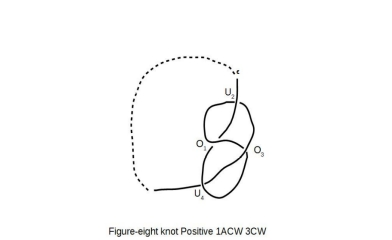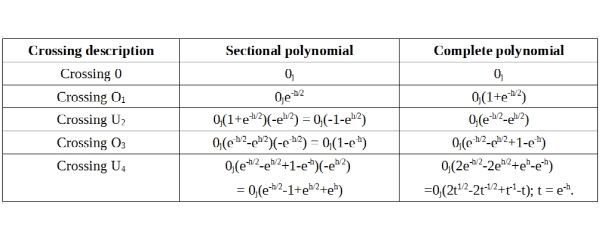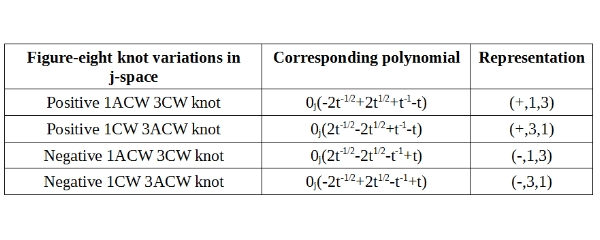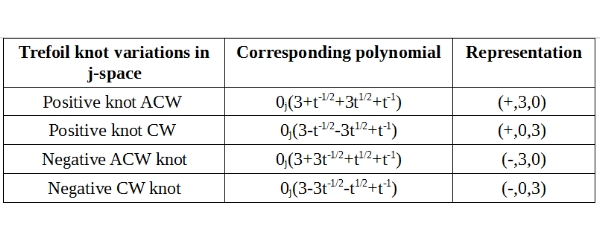Home

The Figure-8 Knot

The knot diagram for the figure-8 knot, is shown as following:The Figure-8 knot shown, begins with Anticlockwise loop for the first crossing written as O1.  The following crossings U2, O3, and U4 are in Clockwise direction.  Hence the notation 1ACW3CW.  We call the knot Positive, as the first crossing is an over-crossing.

The knot polynomial for the figure-8 knot can be written, in the manner similar to the one introduced for Trefoil knot earlier as following:As discussed earlier while drawing the Figure-8 knot, we noticed that the directions of sectional loops, are Clockwise and Anticlockwise both with in the same knot.  In Trefoil knot the direction is exclusively either Clockwise and Anticlockwise.  We therefore need to introduce a representation which along with the knot-polynomial, provides a complete picture to the observer.  An example based on Figure-8 knot shown above, is as following:The knot description consists of the knot polynomial and representation columns.  For example (+, 1, 3) in above table, describes a knot whose first crossing is over-crossing (+).  One of its crossings, is the result of a loop with ACW orientation, whereas three of its crossings are result of loops with ACW orientation.  We can create similar description for Trefoil knot as well, as shown below:In this case, for example, (+, 3, 0) describes a knot, whose first crossing is an over-crossing (+).  This knot has a total of three crossings.  Since this knot is in j-space, the crossings are alternating by default.  All three crossings are the result of loops with ACW orientations, hence the value 3.  There is no loop in this knot with a CW orientation, hence the value 0.

If we compare the Trefoil and Figure-8 knots, we notice the absence of constant in the knot polynomial for Figure-8 knot.  The Trefoil knot in contrast has for example, a value "3" representing the contributions of 0j.  This is an important difference as the absence of contribution of 0j in knot polynomial for figure-8 knot, represents the corresponding physical structure, which has no memory of the initial state.  The structures with no memory of the initial state, are statistical in nature described by random variables.  Thus if we write a knot polynomial which show no contribution of
0j, then it is likely to be of statistical nature.  Such structures can be further reduced by a process similar to symmetry breaking, until contribution of 0j is accounted for, in the knot polynomials.  The most fundamental structure clearly is represented by Trefoil knots, which have three alternating crossings the required minimum to form a knot.

We have shown that knot polynomials written for the discrete measurement space or j-space, allow us to differentiate between the knots which are of statistical nature and those which are of more fundamental nature.  The knots representing a system with the memory of the initial state will have finite contribution from the terms corresponding to 0j.  Whereas the knots showing no contributions from 0j in their respective polynomials,  are of statistical nature.  We can easily write similar polynomials along with representations for higher order alternating knots in j-space, and apply the criterion stated here to determine the type of system they represent.

A fantastic application, will be to apply j-space knot polynomials, to the mechanism used by enzymes to unpack DNAs.  In j-space, enzymes and DNAs both are observers, who are making measurements and being measured at the same time.  Each molecule in a DNA represents a measurement.  Subsequently by stringing together these measurements, a knot polynomial can be written for a specific DNA.  The DNAs represented by knot polynomials with no contribution from 0j term, are more likely to be affected by enzymes and hence possibly more susceptible to the diseases.  Similarly the DNAs represented by very high contributions of 0j term in their knot polynomials, are likely to be much more robust.DNA and Enzymes

Taking the argument further we can possibly also write similar knot polynomials for enzymes in j-space.  If we compare the knot polynomials written for enzymes and DNAs, the enzymes corresponding to the polynomials of lower order (t-n, t+n) and with high contributions of 0j terms, are more likely to succeed in breaking down DNAs corresponding to the polynomials of higher order and with small contributions of 0j terms.  In similar fashion, the DNAs with lower order polynomials and with high contributions from 0j terms, should be immune to the effects of enzymes corresponding to higher order polynomials and with little contributions from 0j terms.

updated 2nd April 2018